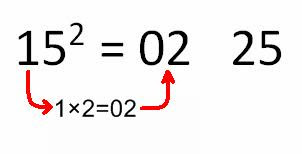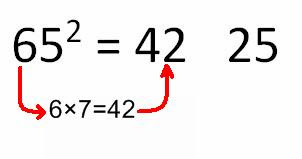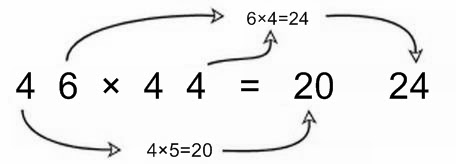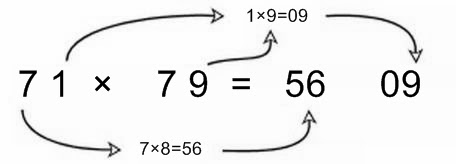# How to Square & Multiply Numbers Quick & Easily - Vedic Mathematics Method

Part 1): A simple and quick method on How to square a number ending with 5.

In this tutorial we are going to learn one simple method of Vedic mathematics to make the square of a number which ends with 5.

Whenever a number ends with 5, definitely the last two digits will be 25. Now the remaining part of the answer can be calculated by multiplying a number with its next. For example if the given number is 35 then first part will be 25 and the remaining part will be 3×4=12. So the answer is 1225.

Example: Find square of 15.Here the last two digits will be 25 and the first two digits are calculated by 1×2=02. So the answer is 225.

Let us take another example for clear understanding.

Example: Find square of 65.

Solution: first part will be 6×7=42. So the answer is 4225.Part 2): Multiplication of two numbers having first digit same and last digits add up to 10.

Example: Multiply 46×44.

Solution:• Bothe numbers having digit 4 same.

• Last digits that are 6 and 4 add up to 10.

• So we just multiply 4×5 =20 for getting first part of the answer.

• Also multiply last digits (6×4=24) for second part of the answer.

• So the answer is 2024.

Example: Multiply 71×79.

Solution:• Bothe numbers having digit 7 same.

• Last digits that are 1 and 9 add up to 10.

• So we just multiply 7×8=56 for getting first part of the answer.

• Also multiply last digits (9×1=09) for second part of the answer.

• So the answer is 5609.

Related articles